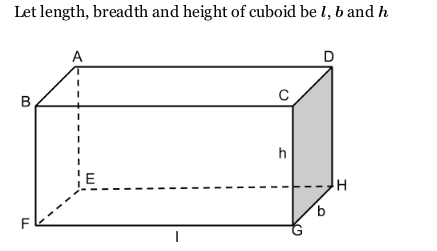# What is total surface area of cuboid?

Solution:

The total surface area (TSA) of a cuboid is the sum of the areas of its 6 faces.A cuboid has six faces.

Area of BFGC and AEHD {each} = $h \times l$

Area of ABFE and CGHD {each} = $b \times h$

Area of ABCD and EFGH (each )=$l \times b$

Therefore, area of all faces =$2(h \times l)+2(b \times h)+2(l \times b)$

=$2(h\times l+b \times h+l \times b)$

=$2(l\times b+b \times h+h \times l)$

It is called total surface area of a cuboid.